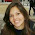## Tuesday, November 23, 2010

### Slope One

Slope One is a simple and efficient type of recommender system. Introduced by Daniel Lemire and Anna Maclachlan in 2005, it involves a simpler idea than the majority of other collaborative filtering implementations. While these usually calculate the similarity between vectors of items using the cosine or the Pearson methods, the Slope One approach recommends items to users based on the average difference in preferences of items.

The main idea of the algorithm is to create a linear relation between items preferences such as the relation F(x) = x + b. The name "Slope One" cames from the fact that here the "x" is multiplied by "1".

It basically calculates the difference between the ratings of items for each user (for every item the user has rated). Then, it creates and average difference (diff) for every pair of items. To make a prediction of the Item A for an User 1 for example, it would get the ratings that User 1 has given to other items and add to it the difference (diff) between each item. With this we could obtain an average.

Being R1B the rating that user 1 has given to item B, DiffAB the difference between ratings of item A and B, and supposing we have 10 items:

Prediction(Item A) for User 1  = (R1B + DiffAB) + (R1C + DiffAC) + ... (R1J + DiffAJ)
10

Example

Suppose that we have a matrix of ratings from users to items. We have an user A that has given an item I the rating 1 and item J the rating 1.5. We also have an user B that has given item I the rating 2.
Based on that, how would slope one calculate the rating from user B to item J?

The algorithm would say that there is a linear relation between the differences of the items. So if the  preference difference of items I and J by user A is 0.5, we would apply this difference to the rating user B has given to I and obtain a rating to J.
Basically , we have

Pref(J) by user B = Pref (I) + Diff I J

Another example:

The prediction from user A to item 3 would be:

Diff (item 3 x item 1) = 3 – 4 => - 1
Diff (item 3 x item 2) = 3 – 3 => 0

3 is the rating given by user A to item 1 and 5 is the rating given to item 2. So we add his given ratings to the diffs.

P(item 3) = [(- 1 + 3) + ( 0 + 5) ] / 2 =>  3.5

Pseudo-Code

Below I present a pseudo-code version of the algorithm. It can be divided in two parts:

The preprocessing phase, in which is calculated the
difference between all item-item preference values

1. for every item i
2.  for every other item j
3.   for every user u expressing preference for both i and j
4.     add the difference in u’s preference for i and j to an average

The prediction part

1. for every item i the user u expresses no preference for
2.  for every item j that user u expresses a preference for
3.   find the average preference difference between j and i
4.   add this diff to u’s preference value for j
5.   add this to a running average
6. return the top items, ranked by these averages

Complexity

In terms of execution, the most expensive phase is the preprocessing one, which can be precomputed. The algorithm is very attractive because its online portion, the prediction phase, is fast.
Its running time does not depend on the number of users, it depends mostly upon the average preference difference between every pair of items. Suppose we have m users and n items, computing the average differences could take up to m n2 time steps. The storing of the diff matrix could also be expensive. It could take up to n(n-1)/2 units of storage.

Useful links:

#### 4 comments:

1.This Blog is really helpful. Thank you so much Ma'am!

1.Glad you liked! :D

2.Hi Ma'am,
Can I use Slope One to predict items to users using data fetched from sql in an asp.net application?
I will realy appriciate If you can provide me with some guidelines. Thank you so much Ma'am.

1.Humm I believe that it could. I think it matters more how is the data than where the data comes from. What data do you intend to use to describe the user's preferences? Can you get some kind of rating there?